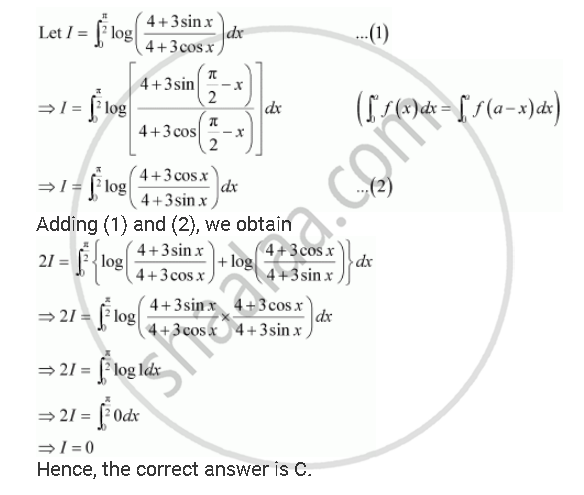Share

Books Shortlist

Choose the Correct The Value of Int_0^(Pi/2) Log ((4+ 3sinx)/(4+3cos X)) Dx is - CBSE (Commerce) Class 12 - Mathematics

ConceptProperties of Definite Integrals

Question

Choose the correct The value of int_0^(pi/2) log  ((4+ 3sinx)/(4+3cos x)) dx is

A. 2

B. 3/4

C. 0

D. -2

SolutionIs there an error in this question or solution?

Video TutorialsVIEW ALL 

Solution Choose the Correct The Value of Int_0^(Pi/2) Log ((4+ 3sinx)/(4+3cos X)) Dx is Concept: Properties of Definite Integrals.
S